Previous Article in Journal
Photomechanical Bending of Azobenzene-Based Photochromic Molecular Fibers
Previous Article in Special Issue
Tunable Sensor Response by Voltage-Control in Biomimetic Hair Flow Sensors
Open AccessArticle

# Pushing the Limits of Electrical Detection of Ultralow Flows in Nanofluidic Channels

byKlaus Mathwig andSerge G. Lemay *
MESA+ Institute for Nanotechnology, University of Twente, PO Box 217, 7500 AE Enschede, The Netherlands
*
Author to whom correspondence should be addressed.
Micromachines 2013, 4(2), 138-148; https://doi.org/10.3390/mi4020138
Received: 11 December 2012 / Revised: 30 January 2013 / Accepted: 20 March 2013 / Published: 2 April 2013

## Abstract

This paper presents improvements in flow detection by electrical cross-correlation spectroscopy. This new technique detects molecular number fluctuations of electrochemically active analyte molecules as they are transported by liquid flow through a nanochannel. The fluctuations are used as a marker of liquid flow as their time of flight in between two consecutive transducers is determined, thereby allowing for the measurement of liquid flow rates in the picoliter-per-minute regime. Here we show an enhanced record-low sensitivity below 1 pL/min by capitalizing on improved electrical instrumentation, an optimized sensor geometry and a smaller channel cross section. We further discuss the impact of sensor geometry on the cross-correlation functions.

## 1. Introduction

Microfabricated flow sensors are used in a wide range of commercial and research applications, their importance being reflected in the vast number of existing sensors and measurement concepts [1,2]. Also in nanofluidic systems, the flow rate is a desirable property to quantify, e.g., when measuring liquid slip or investigating the transport of macromolecules through nanochannels .
We have recently introduced electrochemical cross-correlation spectroscopy as an all-electrical method to detect ultralow picoliter-per-minute flow rates in nanofluidic channels . The basis of this method is nanogap sensors , in which electrochemical active molecules undergo redox cycling between two closely spaced electrodes embedded in opposite walls of a nanochannel. The molecules travel back and forth by diffusion and are repeatedly oxidized and reduced at the electrodes, thereby shuttling electrons across the channel and generating an electrical current. This current is directly proportional to the number of molecules in the nanogap sensor. Since the molecules undergo random thermal Brownian motion, their number fluctuates considerably in time in the small femtoliter detection volume—an effect that is reflected in corresponding fluctuations of the electrical current.
To measure liquid flow, we detect the time of flight of this diffusion noise between two consecutive transducers, as sketched in Figure 1. The fluctuations are measured by recording current-time traces at the top electrodes of both sensors. The time of flight is then extracted by cross-correlation analysis of these traces.
Figure 1. Schematic of the experimental setup. Fluctuations in the number density of electroactive molecules are used as tracers of liquid flow as solution is transported through a nanochannel. The fluctuations are detected electrically by redox cycling and their time of flight between the detectors—or, equivalently, the flow velocity—is then determined by cross correlation analysis of current-time traces (curves in the insets are a schematic illustration).
Figure 1. Schematic of the experimental setup. Fluctuations in the number density of electroactive molecules are used as tracers of liquid flow as solution is transported through a nanochannel. The fluctuations are detected electrically by redox cycling and their time of flight between the detectors—or, equivalently, the flow velocity—is then determined by cross correlation analysis of current-time traces (curves in the insets are a schematic illustration).## 2. Device Fabrication

Nanogap devices were fabricated as described previously  on an oxidized 4-inch silicon wafer. 20 nm thick Pt bottom electrodes were deposited by electron-beam evaporation using a Balzers BAK 600 and patterned by photolithography and a lift-off process using Arch Chemical OIR 907-17 photoresist. The nanochannel was defined as a 55 nm thick Cr layer also deposited and defined by electron beam deposition and photolithography, respectively. After deposition of a 100 nm thick Pt top electrode, the whole structure was buried in a passivating SiN layer deposited by chemical vapor deposition. At both ends of the nanochannel, access holes were etched into the passivation layer by reactive ion etching. Schematic cross sections of the device are shown in Figure 2. It consists of a 202 μm long, 5 μm wide and 55 nm high nanochannel. The electrode pairs at the channel floor and ceiling are 100 μm long and are separated by a 2 μm wide gap.
Liquid flow is generated in the nanochannel by a syringe pump (Harvard Apparatus Pump 11 Pico Plus Elite). Since ultralow flow rates cannot be driven directly in this manner, the pump flow is reduced via Parallel Flow Control . Tubing (TYGON S-54-HL) is connected to the nanofluidic device via an additional microfluidic layer in polydimethylsiloxane (PDMS, Dow Corning Sylgard 184), which was formed by molding on an SU-8/silicon master and bonded to the SiN surface after surface activation in an oxygen plasma. In this layer, four 200 μm long, 5 μm wide and 3 μm high microchannels connect both access holes of the nanochannel. The flow is then divided among the parallel nano- and microchannels according to the ratio of their hydraulic resistances. Since the resistances change cubically with the channel height the nanochannel flow rate is reduced by a factor of approximately 400,000 with respect to the syringe pump flow rate. Micrographs of the device are shown in Figure 2.
Figure 2. (a) Schematic cross section of the device along the longitudinal (top) and lateral axis (bottom). (b) Top view micrograph of a 202 μm long nanofluidic device bonded to a polydimethylsiloxane (PDMS) microchannel layer (only two out of four microchannels running in parallel to the device are shown). (c) Micrograph of the overall chip structure. (d) Photography of a chip bonded to PDMS connected to tubing; electrical contact pads are visible on the right.
Figure 2. (a) Schematic cross section of the device along the longitudinal (top) and lateral axis (bottom). (b) Top view micrograph of a 202 μm long nanofluidic device bonded to a polydimethylsiloxane (PDMS) microchannel layer (only two out of four microchannels running in parallel to the device are shown). (c) Micrograph of the overall chip structure. (d) Photography of a chip bonded to PDMS connected to tubing; electrical contact pads are visible on the right.## 3. Experimental

Ferrocenedimethanol (Fc(MeOH)2 from Acros, diffusion coefficient D = 6.7 × 10−10 m2/s) was chosen as redox-active species and prepared as a 1 mM solution in Milli-Q water with 0.1 M KCl (Sigma-Aldrich) added as background electrolyte together with 5 mM H2SO4 (Sigma-Aldrich) to prevent electrode degradation. Directly before a measurement, the Cr sacrificial layer was removed and the nanochannel was formed by purging the microchannels with a chromium etchant solution (Selectipur, BASF). The redox active solution was then driven through the channel with varying syringe pump flow rates of up to 60 μL/h.
Both the bottom and top electrodes of the device were connected to a CH Instruments 842B bipotentiostat. Both top electrodes were biased at an oxidizing potential of 0.45 V while the bottom electrodes were biased at a reducing potential by short-circuiting them to a Ag/AgCl reference electrode that was connected by tubing downstream of the device. The whole setup except for the bipotentiostat (but including the syringe pump) was shielded from interference in a Faraday cage. Current-time traces of up to 600 s length were recorded at 10 ms sampling intervals at both top electrodes. We used high pass filtering to remove slow instrumental drift (see below).

## 4. Results and Discussion

The times of flight of molecular number concentrations were extracted from the detected current-time traces by cross-correlation analysis : the traces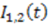exhibit diffusion noisefrom which a normalized cross-correlation function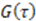is evaluated: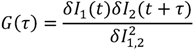peaks at the time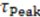, which is—in a first approximation—identical to the time of flight of Fc(MeOH)2 molecules from the center of the first transducer to the center of the second one located 102 μm downstream. In Figure 3, cross-correlation functions are shown as a function of the syringe pump flow rate and compared to analytical functions for one-dimensional drift and diffusion in the same device geometry .
Figure 3. (a) Cross-correlation functions for different syringe pump flow rates determined from current-time traces recorded at both 100 μm long top electrodes of a 202 μm long nanofluidic device. (b) Analytically derived cross-correlation functions for different molecular drift velocities v for the same device geometry and an effective diffusion coefficient of D = 3.4 × 10−10 m2/s.
Figure 3. (a) Cross-correlation functions for different syringe pump flow rates determined from current-time traces recorded at both 100 μm long top electrodes of a 202 μm long nanofluidic device. (b) Analytically derived cross-correlation functions for different molecular drift velocities v for the same device geometry and an effective diffusion coefficient of D = 3.4 × 10−10 m2/s.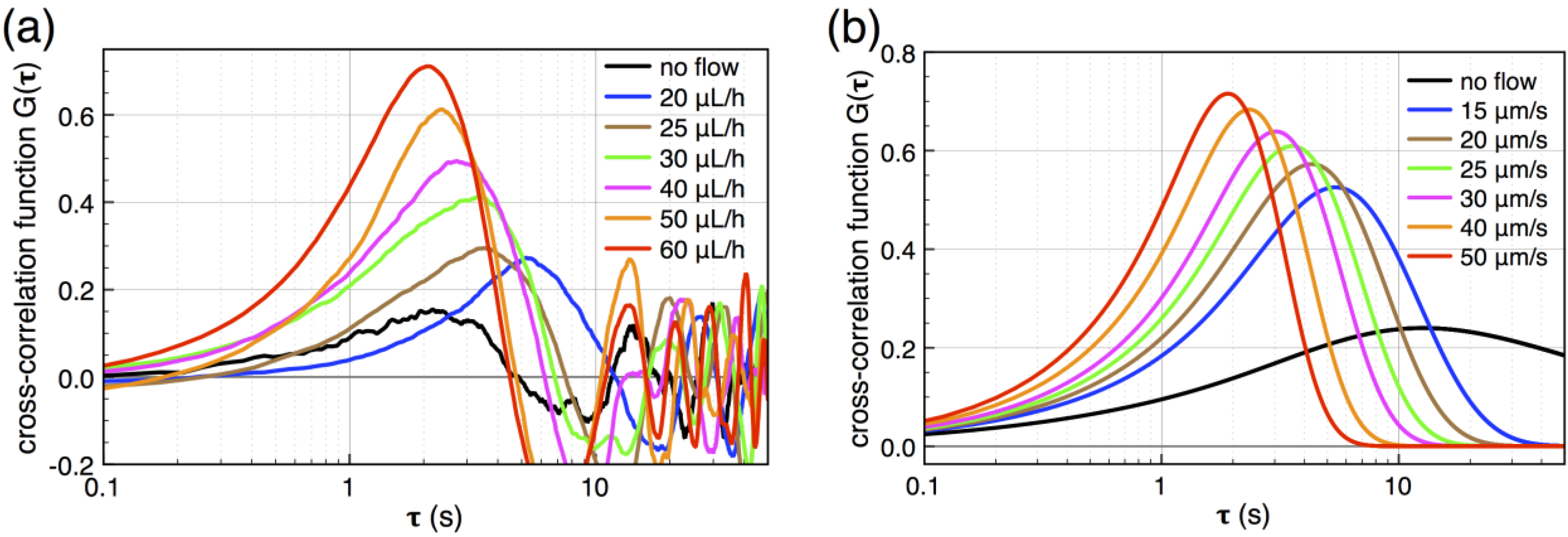The curves are defined exclusively by longitudinal advection and diffusion. The increasing influence of diffusion for decreasing flow rates leads to peak broadening and loss of correlation. It prohibits the detection of times of flight longer than approximately 8 s because the number fluctuations decay diffusively during the flight.
The liquid flow rate in the nanochannel was first determined by multiplying the center-to-center distance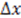of the transducers with the channel cross section and dividing by. It is shown in Figure 4 as a function of pump flow (orange diamonds).
Figure 4. Nanofluidic flow rates as a function of syringe flow. The adjusted experimental data points are corrected for the shift of the peak times as well as for dynamic adsorption. The dashed line’s slope corresponds to the ratio of the micro- and nanochannels’ resistances of 1/400,000.
Figure 4. Nanofluidic flow rates as a function of syringe flow. The adjusted experimental data points are corrected for the shift of the peak times as well as for dynamic adsorption. The dashed line’s slope corresponds to the ratio of the micro- and nanochannels’ resistances of 1/400,000.However, two effects need to be accounted for for a more accurate flow detection. First, due to the high surface-to-volume ratio of the nanochannel of 2 × 107 m−1, the Fc(MeOH)2 molecules undergo pronounced dynamic reversible adsorption at the electrodes and nanochannel walls, which slows down their average transport with respect to the fluid. We estimate the relative number of adsorbed moleculesby stochastic chronoamperometry . Its magnitude exhibits considerable scatter but amounts approximately to= 0.5, i.e., the molecules are slowed down to 50% of the mean liquid flow velocity.
Secondly, the increasing influence of diffusion shifts the cross-correlation peak timeaway from the actual times of flight for slow flow rates. That is, molecules travel faster into the subsequent detector by diffusion than by advection, so thatbecomes smaller than the time of flight. This shift is determined from the known analytical cross-correlation function (see below, ) and is shown in Figure 5a for the molecular diffusion coefficient D = 6.7 × 10−10 m2/s (green curve) and an adjusted effective Deff = 0.5 D, which takes into account the reduction of diffusive transport by the dynamic adsorption. In Figure 5b, this effect is exemplified by analytical cross-correlation functions with a constant time of flight of 4 s and flow rates ranging from 250 μm/s to 10 μm/s (and corresponding electrode lengths of 40 μm to 1000 μm with no gap in between.)
Nanochannel flow rates adjusted for both effects are shown as blue triangles in Figure 4. (The nonlinear decrease in nanochannel flow for higher pump flow rates is caused by PDMS bulging at high pressures .) We are able to detect flow rates below 1 pL/min, which is a factor of ten smaller compared to our previous measurements .
Figure 5. (a) Analytically determined deviation of the cross-correlation function’s peak timefrom the time of flight as a function of flow velocity. The blue curve is corrected for an effectively slower diffusion of the molecules due to dynamic adsorption. Experimental flow rates range from 15 μm/s to 50 μm/s. (b) Cross-correlation functions for a constant time of flight of 4 s but with different molecular velocities v (and corresponding different electrode lengths L or flight paths, respectively).
Figure 5. (a) Analytically determined deviation of the cross-correlation function’s peak timefrom the time of flight as a function of flow velocity. The blue curve is corrected for an effectively slower diffusion of the molecules due to dynamic adsorption. Experimental flow rates range from 15 μm/s to 50 μm/s. (b) Cross-correlation functions for a constant time of flight of 4 s but with different molecular velocities v (and corresponding different electrode lengths L or flight paths, respectively).#### 4.1. Detection Sensitivity

At low flow rates, the sensitivity in flow detection is ultimately limited by diffusive decay of the correlations during the time of flight. In particular, the flow velocity must satisfyfor a pronounced cross-correlation peak to appear [4,10]; at lower flow rates, the signal becomes essentially indistinguishable from that for purely diffusive mass transport.
A second requirement for detection is a sufficiently high signal-to-noise ratio (SNR) of the cross-correlation function. The SNR has been analyzed in detail for Fluorescence Correlation Spectroscopy (FCS) and, because of the close relation between the two methods , this analysis is directly applicable here. For the case of a high number of molecules in the detection region and in the so-called molecular noise limit the SNR is given by [11,12].Here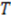is the duration of a current–time trace andis approximately the decay time of the correlations, i.e., the time of flightThis expression for the SNR is valid for the limits, where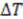is the sampling interval. For the slowest flow reported here, the diffusive noise is not limiting since it still corresponds to SNR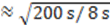= 5.
The counterpart to the “Poisson noise limit” in FCS, in which shot noise in the photon count rate dominates the SNR [11,12], is not observed in electrical correlation spectroscopy. Due to the short (microseconds) diffusive shuttling time across the channel and slow (milliseconds) electronic response times, the number of electrons shuttled per measurement interval is always.
For the high 1 mM analyte concentration with millions of molecules in the detection area, the influence of background noise on the current-time traces is negligible  and plays no significant role for the flow detection.
The increase in sensitivity is achieved for three reasons:
• The nanochannel has a height of 55 nm instead of 130 nm. For the same average flow velocity, the flow rate is simply reduced by the smaller channel cross-section.
• The length of each electrode is increased from 50 μm to 100 μm. As shown below (Figure 6), this increases the signal strength because the correlation in a longer plug of fluid takes more time to decay. However, a longer electrode also has the undesirable effect of decreasing the SNR according to Equation (2),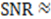(𝑣/𝐿)1/2. For our measurement parameters (T > 100 s, v > 10 μm/s, L = 100 μm, SNR > 10), this is however not limiting.
• Since our method relies on the detection of purely stochastic noise, sensitivity is increased by sampling traces with longer durations T. Our previous instrumentation (Keithley 6430 sub-femtoamp source meters) limited the current-time traces to a length of 25 s (at a fast= 100 s−1 acquisition rate). The instrumentation used here allowed extending the measurement period to up to 600 s at the same sampling interval. Therefore the traces are considerably longer than the decay timeand the SNR is improved.
Diffusion sets the limit of the slowest resolvable velocity in the nanochannel to. However, for our current setup, the sensitivity is ultimately limited by instrumental drift of the measured current of about 0.5% or 1 nA peak-to-peak, most likely caused by temperature drift. This external noise occurs on time scales of up to several 10’s of mHz and can be reduced by high-pass filtering (4th order Butterworth filter) with a f = 100 mHz cutoff frequency. The drift essentially prohibits detection of slower flow rates with corresponding times of flight longer than about 10 s. For longer times, the diffusion noise is lost in the instrumental drift, which occurs at the same time scales but exhibits a larger amplitude.

#### 4.2. Geometric Design Rules

As derived in Reference  and in agreement with the experimental results, the cross-correlation function is given analytically byHere, the upstream electrode is positioned at, and the downstream electrode is located at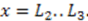Compared to expressing G as a function of one electrode length and a gap length in between the electrodes , the expression shown here more generally allows for asymmetric electrodes as well as ‘negative’ gap lengths, i.e., overlapping electrodes. In Figure 6, the impact of different electrode lengths on the shape of the cross-correlation function is shown for a mean flow velocity of v = 100 μm/s, D = 5 × 10−10 m2/s and no gap between the electrodes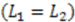. With increasing sensor length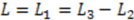the magnitude of the peak in the correlation function increases, which is favorable. The position of the peak however simultaneously shifts to longer times. Therefore, increasing the detector length beyond several hundreds of μm will not deliver a more sensitive flow detection, since long times of flight are obscured by instrumental drift. Furthermore, the pressure necessary to drive a given flow increases linearly with the channel length and can then exceed 1 bar for a typical channel cross-section, which is often impractically high.
Figure 6. Analytical cross-correlations function as a function of electrode length L for symmetric transducers for a constant velocity v = 100 μm/s, D = 5 × 10−10 m2/s and no gap in between the consecutive sensors. The cross-correlation peaks are more pronounced with increasing L and shift to longer.
Figure 6. Analytical cross-correlations function as a function of electrode length L for symmetric transducers for a constant velocity v = 100 μm/s, D = 5 × 10−10 m2/s and no gap in between the consecutive sensors. The cross-correlation peaks are more pronounced with increasing L and shift to longer.Increasing the distance between the two transducers leads to less pronounced cross-correlation peaks as the molecular number fluctuations have more time to decay diffusively during a longer flight. The influence of varying this gap is shown in Figure 7 for a flow rate of v = 100 μm/s and 100 μm long electrodes. The cross-correlation peak is most pronounced in the limit of a gap of0 μm (red curve). For longer flight paths, the influence of diffusion during a longer flight becomes more dominant and the peaks are shallower (orange curves).
Figure 7. Analytical correlation functions for a varying separation distance L2 - L1 in between consecutive electrodes for v = 100 μm/s, D = 5 × 10−10 m2/s,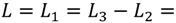100 μm. Green curve: Autocorrelation, which corresponds to L2 = 0; blue curves: intermediate auto-cross-correlation for a gap length L2 - L1 = −75 μm, −50 μm, −25 μm; red, orange, purple curves: cross-correlation with L2 - L1 = 0 μm…1000 μm.
Figure 7. Analytical correlation functions for a varying separation distance L2 - L1 in between consecutive electrodes for v = 100 μm/s, D = 5 × 10−10 m2/s,100 μm. Green curve: Autocorrelation, which corresponds to L2 = 0; blue curves: intermediate auto-cross-correlation for a gap length L2 - L1 = −75 μm, −50 μm, −25 μm; red, orange, purple curves: cross-correlation with L2 - L1 = 0 μm…1000 μm.For completeness, we note that experimentally also overlapping detection areas (i.e., “negative gap lengths”) are feasible: To allow for redox cycling to take place, one transducer consists of two opposing electrodes. Since every molecule that is oxidized on one electrode will be reduced at the opposing one, the current-time traces of these electrodes are perfectly anticorrelated , and (anti-) cross-correlating these two traces is identical to determining the autocorrelation function (green curve) of just one trace [4,14]. Therefore, anti-correlating traces of only partially overlapping top- and bottom electrodes leads to correlation functions in between auto- and cross-correlation (blue curves). While these intermediate “anti-auto-cross-correlation” functions are not useful in themselves, we do see applications for asymmetric top and bottom electrodes in future experiments: By fabricating slightly longer electrodes at the downstream end of a transducer it is possible to insure that either only reduced or oxidized molecules leave the transducer instead of an equal mixture for a symmetric electrode pair. Also, if one electrode overlaps with two opposing electrodes and is kept floating electrically, an intermediate potential of the two opposing potentials is induced, thereby allowing for experiments analogous to induced-charge electrokinetics .
We finally note that, for sufficiently long gaps (<, purple curve in Figure 7), the cross-correlation functions exhibit a Gaussian shape, and Equation (3) reduces towith a center-to-center distance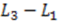for electrodes of equal length, a standard deviation ofand a mean or time of flight ofSuch a long flight path between two detectors may allow the “chromatographical” discrimination of two different redox active species if they move at different velocities due to a difference in adsorption.

## 5. Conclusion and Outlook

We have used all-electrical cross-correlation spectroscopy of mesoscopic number fluctuations to detect record-low liquid flow rates below 1 pL/min in electrochemical nanogap sensors. This is comparable to the sensitivity recently achieved by the related method of determining the autocorrelation function of a single current-time trace as a function of flow in a 100 μm long and 50 nm high sensor .
We emphasize that the determined nanochannel flow rates are subject to error due to uncertainties in channel height,, and adsorption. Thus, while detection in the sub-picoliter-per-minute range is possible, we do not claim a precision measurement in this regime.
Our method is the direct electrical analogue to optical fluorescence correlation spectroscopy . Therefore we envision its application not exclusively for flow detection and have discussed the dependence of the correlation function on device geometry more generally. The method could become a tool to investigate properties of smallest quantities of analyte molecules such as adsorption, concentration or electrochemical reaction kinetics.

## Acknowledgments

We gratefully acknowledge financial support from the Netherlands Organization for Scientific Research (NWO) and the European Research Council (ERC). We thank S. Kang for help with device fabrication and D. Mampallil for useful discussions.

## References

1. Kuo, J.T.W.; Yu, L.; Meng, E. Micromachined thermal flow sensors–A review. Micromachines 2012, 3, 550–573. [Google Scholar] [CrossRef]
2. Silvestri, S.; Schena, E. Micromachined flow sensors in biomedical applications. Micromachines 2012, 3, 225–243. [Google Scholar] [CrossRef]
3. Westin, K.J.A.; Choi, C.-H.; Breuer, K.S. A novel system for measuring liquid flow rates with nanoliter per minute resolution. Exp. Fluids 2003, 34, 635–642. [Google Scholar] [CrossRef]
4. Mathwig, K.; Mampallil, D.; Kang, S.; Lemay, S.G. Electrical cross-correlation spectroscopy: Measuring picoliter-per-minute flows in nanochannels. Phys. Rev. Lett. 2012, 109. [Google Scholar] [CrossRef]
5. Zevenbergen, M.A.G.; Wolfrum, B.L.; Goluch, E.D.; Singh, P.S.; Lemay, S.G. Fast electron transfer kinetics probed in nanofluidic channels. J. Am. Chem. Soc. 2009, 131, 11471–11477. [Google Scholar]
6. Kang, S.; Mathwig, K.; Lemay, S.G. Response time of nanofluidic electrochemical sensors. Lab Chip 2012, 12, 1262–1267. [Google Scholar] [CrossRef]
7. Liang, H.; Nam, W.J.; Fonash, S.J. A Novell Parallel Flow Control (PFC) System for Syringe Driven Nanofluidics. In Proceedings of the NSTI Nanotechnology Conference and Trade Show, Boston, MA, USA, 1–5 June 2008; Volume 3, pp. 281–283.
8. Singh, P.S.; Chan, H.-S.M.; Kang, S.; Lemay, S.G. Stochastic amperometric fluctuations as a prove for dynamic adsorption in nanofluidic electrochemical systems. J. Am. Chem. Soc. 2011, 133, 18289–18295. [Google Scholar]
9. Gervais, T.; Eli-Ali, J.; Günther, A.; Jensen, K.F. Flow-induced deformation of shallow microfluidic channels. Lab Chip 2006, 6, 500–507. [Google Scholar] [CrossRef]
10. Singh, P.S.; Kätelhön, E.; Mathwig, K.; Wolfrum, B.; Lemay, S.G. Stochasticity in single-molecule nanoelectrochemistry: Origins, consequences, and solutions. ACS Nano 2012, 6, 9662–9671. [Google Scholar] [CrossRef]
11. Koppel, D.E. Statistical accuracy in fluorescence correlation spectroscopy. Phys. Rev. A 1974, 10, 1938–1945. [Google Scholar] [CrossRef]
12. Kask, P.; Günther, R.; Axhausen, P. Statistical accuracy in fluorescence fluctuation experiments. Eur. Biophys. J. 1997, 25, 163–169. [Google Scholar] [CrossRef]
13. Zevenbergen, M.A.G.; Singh, P.S.; Goluch, E.D.; Wolfrum, B.L.; Lemay, S.G. Electrochemical correlation spectroscopy in nanofluidic cavities. Anal. Chem. 2009, 81, 8203–8212. [Google Scholar] [CrossRef]
14. Mathwig, K.; Mampallil, D.; Kang, S.; Lemay, S.G. Detection of Sub-Picoliter-per-Minute Flows by Electrochemical Autocorrelation Spectroscopy. In Proceedings of the 16th International Conference on Miniaturized Systems for Chemistry and Life Sciences (MicroTAS), Okinawa, Japan, 28 October–1 November 2012; pp. 28–30.
15. Bazant, M.Z.; Squires, T.M. Induced-charge electrokinetic phenomena. Curr. Opin. Colloid Interface Sci. 2010, 15, 203–213. [Google Scholar] [CrossRef]
16. Magde, D.; Webb, W.W.; Elson, E.L. Fluorescence correlation spectroscopy. III. Uniform translation and laminar flow. Biopolymers 1978, 17, 361–376. [Google Scholar] [CrossRef]